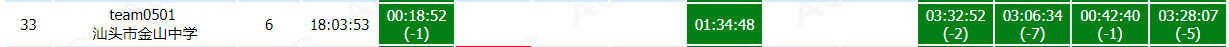2018 Multi-University Training Contest 7 比赛记录Sakits 8月 13, 2018# A. Age of Moyu

### solved by reek. 00:18:52 (-1)

据说是个假题啊…STD也错了，好多写正解的T掉了…
胡爷爷写的是bfs+dfs扩展，感觉没什么太大问题，只要一条边不扩展多次就行了。
这题是个ARC E题原题，正解是拆点。从一种颜色的边到另一种颜色的边代价为$1$，可以看成是地铁站换乘，将一个点新增连接的边的颜色个点，代表着这些线路的站点，原来的点当作一个换乘站，下车不需要代价，但是从换乘站到其他线路的起点需要$1$的代价，就完美解决了边权的问题，最多只会新增$M\times 2$个点。
直接跑dij会带个log，其实可以bfs用双端队列，边权为$0$的扩展塞到队头，为$1$的塞到队尾就可以做到线性复杂度了。

# E. GuGuFishtion

### solved by reek. 01:34:48 (+)

这题貌似有很多做法，这种gcd计数的用容斥当然是最简单的了…
可以一眼看出下面这个显然的变换：

$\sum_{a=1}^n\sum_{b=1}^m\frac{\varphi(ab)}{\varphi(a)\varphi(b)} \Rightarrow \sum_{a=1}^n\sum_{b=1}^m\frac{gcd(a,b)}{\varphi(gcd(a,b))}$

这个式子直接容斥求一波$gcd(a,b)$的出现次数就可以得到答案了。。。
当然莫反随手推一推也出来了，胡爷爷写的就是莫反：

\begin{aligned} ans&=\sum_{a=1}^n\sum_{b=1}^m\sum_{d=1}^n[(i,j)=d]\frac{d}{\varphi(d)}\\ &=\sum_{d=1}^n\sum_{a=1}^{\left \lfloor \frac{n}{d} \right \rfloor}\sum_{b=1}^{\left \lfloor \frac{m}{d} \right \rfloor}[(i,j)=1]\frac{d}{\varphi(d)}\\ &=\sum_{d=1}^n\sum_{a=1}^{\left \lfloor \frac{n}{d} \right \rfloor}\sum_{b=1}^{\left \lfloor \frac{m}{d} \right \rfloor}\sum_{k|(i,j)}\mu(k)\frac{d}{\varphi(d)}\\ &=\sum_{k=1}^n\sum_{d=1}^n\sum_{a=1}^{\left \lfloor \frac{n}{dk} \right \rfloor}\sum_{b=1}^{\left \lfloor \frac{m}{dk} \right \rfloor}\mu(k)\frac{d}{\varphi(d)}\\ &=\sum_{T=1}^n\sum_{d|T}\mu(\frac{T}{d})\frac{d}{\varphi(d)} \end{aligned}

然后就完了。

# H. Traffic Network in Numazu

### solved by reek. 03:32:52 (-2)

这道题打到一半被胡爷爷抢去写了，骗我去写最后一题，还跟我说了个假做法…
带修环套树最短路。。。这题我本来打的是环上用BIT维护顺逆时针走法，树上树剖伺候就好了。
胡爷爷写的是断边树剖，强制经过和不经过分类即可。

# I. Tree

### solved by DriverLao. 03:06:34 (-7)

树上的弹飞绵羊…
有点卡常，这题过的人好少233333

# J. Sequence

### solved by Sakits. 00:42:40 (-1)

整除只有根号段，按这个分段矩乘就好了，有一点细节。
我tmd居然是矩乘板子没1ll*给爆了一发。